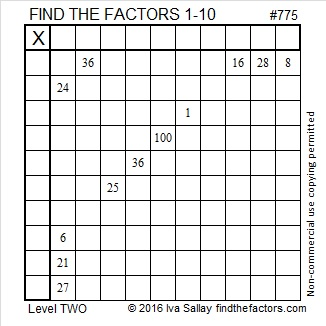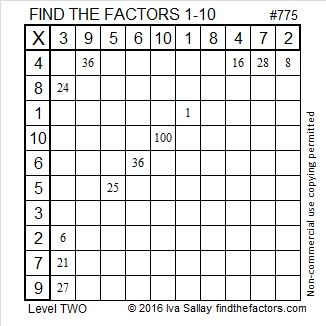# 775 and Level 2

• 775 is a composite number.
• Prime factorization: 775 = 5 x 5 x 31, which can be written 775 = (5^2) x 31
• The exponents in the prime factorization are 2 and 1. Adding one to each and multiplying we get (2 + 1)(1 + 1) = 3 x 2  = 6. Therefore 775 has exactly 6 factors.
• Factors of 775: 1, 5, 25, 31, 155, 775
• Factor pairs: 775 = 1 x 775, 5 x 155, or 25 x 31
• Taking the factor pair with the largest square number factor, we get √775 = (√25)(√31) = 5√31 ≈ 27.83882181.Here’s today’s factoring puzzle:Print the puzzles or type the solution on this excel file: 10-factors-2016

——————————–

Here is more about the number 775:

775 is part of several Pythagorean triples including two that are primitive:

• 168-775-793 (Primitive)
• 775-1860-2015
• 775-9672-9703
• 775-12000-12025
• 775-300312-300313 (Primitive)

775 is palindrome PP in BASE 30 (P = 25 base 10). Note that 25(30) +25(1) = 775.

775 is also the sum of three triangular numbers 9 different ways:

1. 3+276+ 496
2. 6+28+741
3. 6+66+703
4. 10+300+465
5. 15-325-435
6. 36+36+703
7. 78+136+561
8. 171+253+351
9. 120+190+465

——————————–This site uses Akismet to reduce spam. Learn how your comment data is processed.